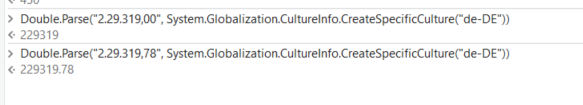# Conversion from string to type 'Double' is not valid

Hii Team,

We are using this code to sum up a column value but are getting this exception
Conversion from string to type ‘Double’ is not valid.

Code Used
((CDbl(((dt_data.AsEnumerable.Where(Function(x)x(“Group account”).ToString.Equals(“680”)).Sum(Function(x)CDbl(x(inconfig(“Month_Column”).ToString).ToString))).ToString))*(-1)).ToString)

The Month Column the values are present in Below Format
2.29.319,00
8.89.616,88
62.66.662,88

Thanks & Regards
@anmita

Hi @anmita

Since the month column data contains characters like `.` and `,`, the exception will be thrown by the target of an invocation. You can try using the following query for the same operation:

`((CDbl(((dt_data.AsEnumerable.Where(Function(x) x("Group account").ToString.Equals("680")).Sum(Function(x) CDbl(x(inconfig("Month_Column").ToString.Replace(".", "").Replace(",", "."))).ToString))).ToString)) * -1).ToString)`

Hope this helps,
Best Regards.

Hii @arjunshenoy

Are there any other alternatives to it

We can correct parse it along with Cultureinfo@ppr thanks for the prompt response

How can we incorporate it into this code
((CDbl(((dt_data.AsEnumerable.Where(Function(x)x(“Group account”).ToString.Equals(“680”)).Sum(Function(x)CDbl(x(inconfig(“Month_Column”).ToString).ToString))).ToString))*(-1)).ToString)

Have tried the method you suggested by incorporating it in into the above code but that was not giving the output

we do have some doubts on your over all LINQ
whenever CDbl is used, just use this other conversion approach
e.g

``````Double.Parse(x(inconfig(“Month_Column”).ToString, System.Globalization.CultureInfo.CreateSpecificCulture("de-DE"))
``````

give a try at:

``````(From d in dt_data.AsEnumerable
Where d("Group account").ToString.Trim.Equals("680")
Let sv = d(inconfig("Month_Column").ToString).ToString.Trim
Let dv = Double.Parse(sv , System.Globalization.CultureInfo.CreateSpecificCulture("de-DE"))
Select x = dv).Sum(Function (x) x)*-1
``````

Hii @ppr

Have tried changing the format as well as the resolutions mentioned its still not working though

We might have to explore other alternative

we do have a set of options in place when parsing string to double. But we dont know what exactly you have done, facing which error and what for values are processed.

So we would recommend to more analyse the root cause as given options where proofed e.g. within immediate panel success check

Understanding the 6 Debugging Panels of UiPath in the easiest way possible! - News / Tutorials - UiPath Community Forum

Thanks for providing Suggestions

Have added the code in Below format

(Double.Parse((CDbl(((dt_data.AsEnumerable.Where(Function(x)x(“Group account”).ToString.Equals(“680”)).Sum(Function(x)CDbl(x(inconfig(“Month_Column”).ToString))))))*(-1)).ToString,System.Globalization.CultureInfo.CreateSpecificCulture(“de-DE”))).ToString

So its giving the below Exception
Conversion from String “10.89.68,06” to Type double is Not Valid

Hi @anmita

To overcome this issue, you need to remove the thousands separators and replace the decimal separators with a period (“.”) before converting the values to double.

Here’s an updated version of the code that incorporates these changes:-
((CDbl(dt_data.AsEnumerable.Where(Function(x) x(“Group account”).ToString.Equals(“680”)).Sum(Function(x) CDbl(x(inconfig(“Month_Column”).ToString).ToString.Replace(“.”, “”).Replace(“,”, “.”))))*(-1)).ToString)

Thanks!!